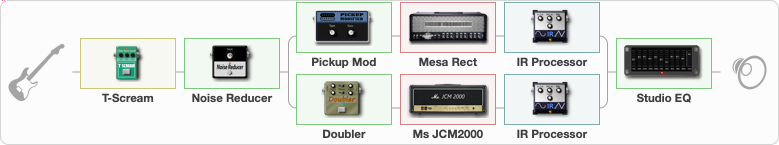# KH AND JUSTICE FOR ALL

Discussion in 'ToneLib-GFX presets' started by grigoriy0192873, Mar 20, 2023.

1. ### grigoriy0192873Active Member

KH AND JUSTICE FOR ALL

Preset name: KH AND JUSTICE FOR ALL

Effects chain:Effect: "T-Scream" (Overdrive / Distortion), active - "yes"
{
"Drive" = 0
"Tone" = 80
"Level" = 100
}

Effect: "Noise Reducer" (Dynamics / Filter), active - "yes"
{
"Sens" = 66
"Mode" = Hard
}

Effect: "Splitter" (Dynamics / Filter)
{
"A-Bypass" = Off
"A-Pan" = -100
"A-Level" = 55
"B-Bypass" = Off
"B-Pan" = 100
"B-Level" = 55
"Width" = 100

'A' branch:
{

Effect: "Pickup Mod" (Dynamics / Filter), active - "yes"
{
"Mode" = Phase Out
"Tone" = 50
}

Effect: "Mesa Rect" (Amp simulators), active - "yes"
{
"Gain" = 45
"Bass" = 65
"Middle" = 32
"Treble" = 86
"Presence" = 70
"Master" = 62
"Level (dB)" = 0
}

Effect: "IR Processor" (Cabinets), active - "yes"
{
"IR" = tolive2-001
"Low Cut (Hz)" = 50
"Hi Cut (kHz)" = 9.0
"Mix" = 100
"Level (dB)" = 0
}
}
'B' branch:
{

Effect: "Doubler" (Dynamics / Filter), active - "yes"
{
"A-Dly" = 67
"A-Inv" = On
"A-Level" = 100
"B-Dly" = 43
"B-Inv" = Off
"B-Level" = 100
}

Effect: "Ms JCM2000" (Amp simulators), active - "yes"
{
"Gain" = 45
"Bass" = 65
"Middle" = 29
"Treble" = 77
"Presence" = 70
"Master" = 51
"Level (dB)" = -1
}

Effect: "IR Processor" (Cabinets), active - "yes"
{
"IR" = justice_R_IR
"Low Cut (Hz)" = 50
"Hi Cut (kHz)" = 9.0
"Mix" = 100
"Level (dB)" = 0
}
}
}

Effect: "Studio EQ" (Dynamics / Filter), active - "yes"
{
"31 Hz" = 0
"62 Hz" = 0
"125 Hz" = 5
"250 Hz" = -2
"500 Hz" = -3
"1 kHz" = 6
"2 kHz" = 4
"4 kHz" = 1
"8 kHz" = 0
"16 kHz" = 0
"Level (dB)" = 0
}

Note: You will need to download and install the ToneLib-GFX software to use the preset.

#### Attached Files:

File size:
8.5 KB
Views:
1,838
• ###### KH_AND_JUSTICE_FOR_ALL.tlgfx
File size:
8.5 KB
Views:
8
Maksim Gaintsev, rst131, Okan and 9 others like this.